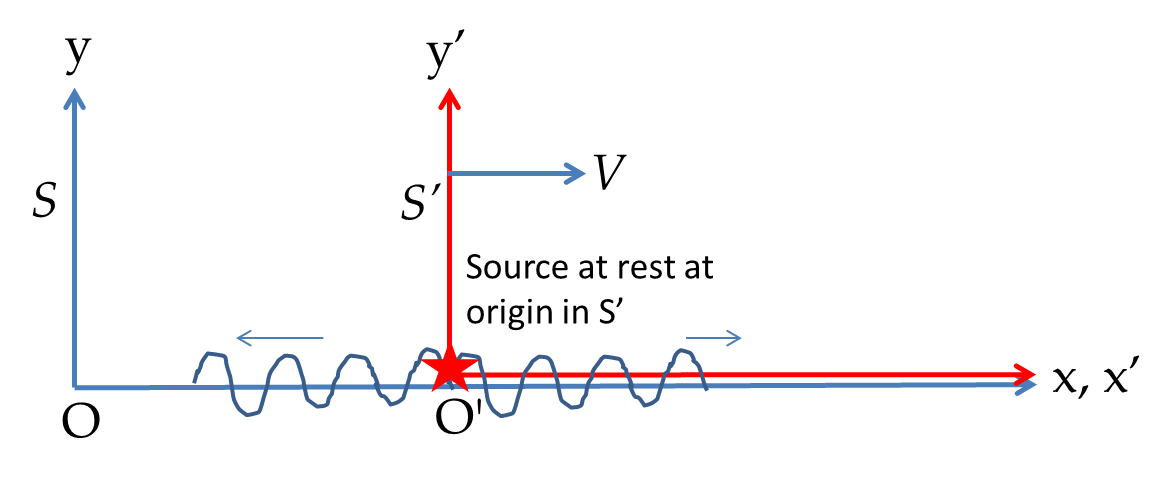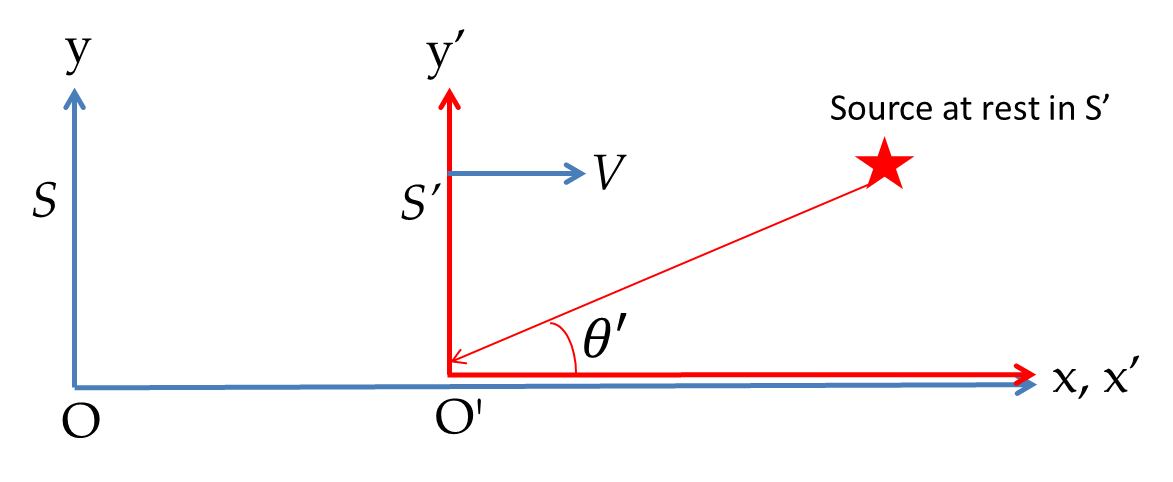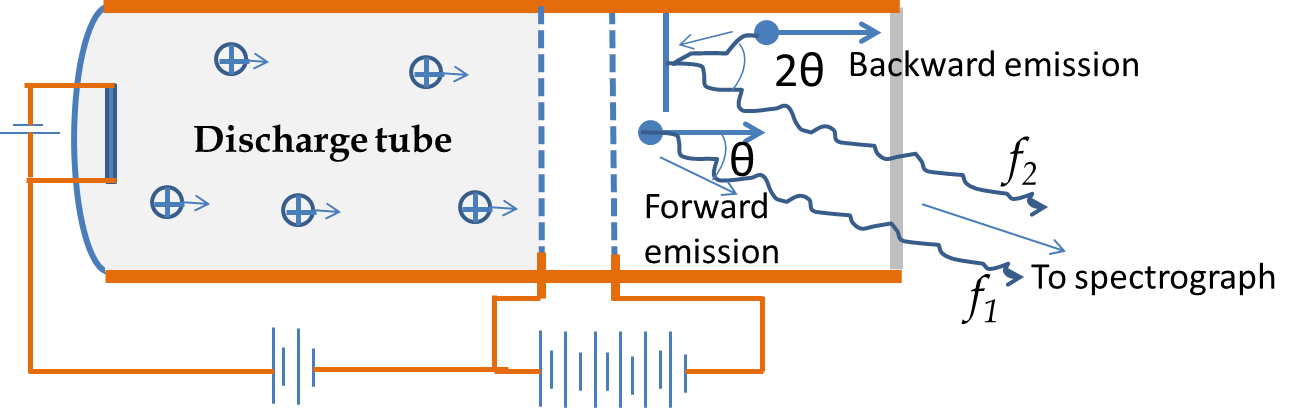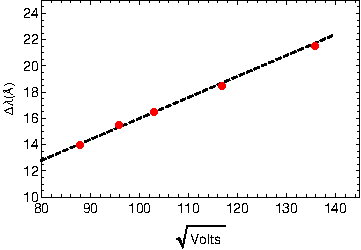## Section52.11Relativistic Doppler Effect

The non-relativistic Doppler effect shows the effect is different when the source is moving and when the observer is moving. Thus, using the non-relativistic Doppler effect it is possible to tell which one of the two frames, the source frame or the observer frame, is moving even though they may be moving at uniform velocity with respect to the medium. This would not happen if you do not have the medium which takes part in the motion of sound waves.

In the case of light waves no medium is necessary. When we work out the frequency of light observed by a detector the shift in frequency is same whether the source is moving or the observer is moving - the effect depends only on the relative motion of the source and the observer. Once again, let $f_0$ be the frequency of the emitted light in the rest frame of the emitter and $f$ be the frequency of the light detected regardless of the state of motion of the detector. Then, we will find below that the Doppler effect, now called relativistic Doppler effect, give the following relation.

\begin{equation} f = f_0\: \sqrt{\dfrac{1- V/c}{1+V/c}}\ \ \ \left\{ \begin{array}{l} \textrm{Observer receding: } V \gt 0\\ \textrm{Observer approaching: } V \lt 0 \end{array} \right.\tag{52.11.1} \end{equation}

To derive this result we will look at a plane wave source traveling along the $x$-axis. To study a moving source we will place the source at the origin of the $\text{S}^{\:\prime}$ frame which moves relative to the S frame towards the positive $x$-axis with speed $V$ as before as shown in Figure 52.11.1.Figure 52.11.1. To calculate the Doppler effect we place the source of sinusoidal wave at rest at the origin of the $\text{S}^{\:\prime}$ frame.

The wave emitted by the source will be traveling towards the negative $x$-axis to reach the detector at the origin of the S frame which is to the left of the origin of the source at the origin of $\text{S}^{\:\prime}$ frame for $t>0\text{.}$ Therefore, the wave at the detector has the following wave function at points on the $x'$-axis in the $\text{S}^{\:\prime}$ frame.

\begin{equation} \psi^{\prime} (x',t') = \psi_0^{\prime} \cos\left( \dfrac{2\pi}{\lambda_0} x' + 2\pi f_0 t'\right). \label{eq-wave-s-prime}\tag{52.11.2} \end{equation}

The Lorentz transformation gives the following relations among the coordinates of S and $\text{S}^{\:\prime}$ frame.

\begin{equation} t' = \gamma \left( t-\dfrac{V}{c^2}\:x \right), x' = \gamma\left(x - V t\right), y' = y, z' = z.\tag{52.11.3} \end{equation}

Now, we express $x'$ and $t'$ in Eq. (52.11.2) in terms of $x$ and $t$ and collect terms in the argument of cosine as a term multiplying $x$ and another term multiplying $t\text{.}$

\begin{equation} \psi^{\prime} (x,t) = \psi_0^{\prime}\cos\left[ 2\pi\gamma \left( \dfrac{1}{\lambda_0} - \dfrac{V f_0}{c^2} \right)\: x + 2\pi\gamma \left(f_0 - \dfrac{V}{\lambda_0}\right) t\right]. \label{eq-wave-s-prime-1}\tag{52.11.4} \end{equation}

In the S frame the wave will be represented by

\begin{equation} \psi(x,t) = \psi_0\cos\left( \dfrac{2\pi}{\lambda_0} x + 2\pi f_0 t\right). \label{eq-wave-s-prime-1a}\tag{52.11.5} \end{equation}

We can ignore the amplitudes and look at the phase to determine the frequency of the wave in the S frame in relation to the frequency of the same wave in the $\text{S}^{\:\prime}$ frame. Equating the factor that multiplies $2\pi t$ in the argument of the cosine function in Eqs. (52.11.4) and (52.11.5) gives the desired relation of the frequency detected in the lab (S) frame in terms of the frequency in the rest ($\text{S}^{\:\prime}$) frame.

\begin{equation} f = \gamma\left( f_0 - \dfrac{V}{\lambda_0}\right). \label{eq-wave-s-prime-2}\tag{52.11.6} \end{equation}

We can write the right-side of this equation in terms of $f_0$ by noting that $f_0\lambda_0 = c\text{,}$ where $c$ is the speed of light. Thus,

\begin{equation} f = f_0\: \sqrt{\dfrac{1- V/c}{1+V/c}}\ \ \ \left\{ \begin{array}{l} \textrm{Observer receding: } V>0\\ \textrm{Observer approaching: } V \lt 0 \end{array} \right.\label{long-doppler-effect}\tag{52.11.7} \end{equation}

By using fundamentl wave equation relating wavelength and frequency, $f = c/\lambda\text{,}$ we can write these for wavelengths in the two frames.

\begin{equation} \lambda = \lambda_0\: \sqrt{\dfrac{1+V/c}{1- V/c}}\ \ \ \left\{ \begin{array}{l} \textrm{Observer receding: } V \gt 0\\ \textrm{Observer approaching: } V \lt 0 \end{array} \right.\label{long-doppler-effect-wavelength}\tag{52.11.8} \end{equation}

The spectrum of light from radio galaxy 8C1435+635 is shifted in wavelength considerably from what you see when emitting atoms are at rest or moving slowly. For instance, the Lyman $\alpha$ line from hydrogen atom spectrum is observed at 639.2 nm instead of 121.6 nm observed in lab. Since the wavelength is increased, which is same as frequency decreased, the galaxy must be receding with respect to us. Suppose, the galaxy is receding directly away from us, what will be the speed of recession?

Hint

Use Doppler shift formula.

$4.25.$

Solution

We use the Doppler formula in the wavelength form.

\begin{equation*} \frac{c}{\lambda} = \frac{c}{\lambda_0}\: \sqrt{\dfrac{1- V/c}{1+V/c}} \end{equation*}

Rearranging we find

\begin{equation*} \frac{1+V/c}{1-V/c} = (\lambda/\lambda_0)^2 = (639.2/121.6)^2 = 27.6. \end{equation*}

Therefore,

\begin{equation*} V = \left(\frac{27.6-1}{27.6+1} \right)\: c = 0.93\:c. \end{equation*}

This says that the radial velocity of the galaxy is 93\% of the speed of light. In Astronomy, one defines a related quiantity call the redshift or $z$ of recession by

\begin{equation*} z = \frac{\Delta \lambda}{\lambda_0}. \end{equation*}

This galxy has $z$ of

\begin{equation*} z = \frac{638.2\:\textrm{nm} - 121.6\:\textrm{nm}}{121.6\:\textrm{nm}} = 4.25. \end{equation*}

### Subsection52.11.1Doppler Effect and Aberration

In the last subsection we studied the relativistic Doppler effect for the relative motion along the line joining the source and the observer. Now, we suppose the source of the wave is in the $xy$-plane and is at rest in the $\text{S}^{\:\prime}$ frame at an angle $\theta'$ in the first quadrant from the origin and counterclockwise from the $x'$-axis.Figure 52.11.3. To calculate the aberration we place the source of sinusoidal wave at rest in the $\text{S}^{\:\prime}$ frame in the direction $\theta^{\prime}\text{.}$

The propagation vector for the ray from the source will be

\begin{equation} \vec k = \dfrac{2\pi}{\lambda_0}\: \left(-\cos\theta'\:\hat i -\sin\theta'\:\hat j \right),\tag{52.11.9} \end{equation}

where $k$ is the wavenumber, and $\hat i$ and $\hat j$ are unit vectors towards the positive $x'$ and $y'$ axes respectively. The wavenumber $k$ is related to the wavelength $\lambda$ by $k = 2\pi/\lambda\text{.}$ Using a general form for oscillating part of plane waves, $\sim \cos(\vec k\cdot \vec r - 2\pi f t)\text{,}$ the wave function for this wave will be

\begin{equation} \psi^{\prime} (x',t') = \psi_0^{\prime}\cos\left[ \dfrac{2\pi}{\lambda_0} (-x'\cos\theta' - y'\sin\theta') - 2\pi f_0 t'\right]. \label{eq-wave-arbitrary-s-prime}\tag{52.11.10} \end{equation}

Now, we express $x'\text{,}$ $y'\text{,}$ and $t'$ in Eq. (52.11.2) in terms of $x\text{,}$ $y\text{,}$ and $t$ using the Lorentz transformations, and collect terms in the argument of cosine as a term multiplying $x\text{,}$ a term multiplying $y\text{,}$ and another term multiplying $t\text{.}$ We also use $\lambda_0 f_0 = c$ to simplify.

\begin{align*} \psi^{\prime} (x,t) = \psi_0^{\prime} \amp \cos\left[ 2\pi\gamma \dfrac{1}{\lambda_0}\left( -\cos\theta' + \dfrac{V }{c} \right)\: x \right.\\ \amp - \left. 2\pi\dfrac{\sin\theta'}{\lambda_0}\: y - 2\pi\gamma f_0\left(1 - \dfrac{V}{c}\: \cos\theta'\right)\: t\right]. \end{align*}

The detector/observer at the origin of the S frame will find this wave coming from the direction $\theta$ and assign the following wave function.

\begin{equation} \psi (x,t) = \psi_0 \cos\left[ \dfrac{2\pi}{\lambda} (x\cos\theta + y\sin\theta) - 2\pi f t\right]. \label{eq-wave-arbitrary-s-prime-2}\tag{52.11.11} \end{equation}

Now, we equate the coefficients of $x\text{,}$ $y\text{,}$ and $t$ in the argument of cosine in the last two equations to obtain

\begin{align} \amp \dfrac{\cos\theta}{\lambda} = \gamma \dfrac{1}{\lambda_0}\left( -\cos\theta' + \dfrac{V }{c} \right) \label{eq-wave-arbitrary-s-prime-3a}\tag{52.11.12}\\ \amp \dfrac{\sin\theta}{\lambda} = \dfrac{\sin\theta'}{\lambda_0}\label{eq-wave-arbitrary-s-prime-3b}\tag{52.11.13}\\ \amp f = \gamma\left(1 - \dfrac{V}{c}\: \cos\theta'\right)\: f_0 \label{eq-wave-arbitrary-s-prime-3c}\tag{52.11.14} \end{align}

We can eliminate $\cos\theta'$ in Eq. (52.11.14) in favor of $\cos\theta\text{.}$ This gives the Doppler shift from a source whose direction is $\theta$ in the frame of the observer while the source is moving at velocity in the direction of $\theta = 0\text{,}$ i.e., the positive $x$-axis.

\begin{equation} f = \left[\frac{1-(V/c)\cos\theta}{\sqrt{1-V^2/c^2}}\right]\ f_0. \label{eq-wave-arbitrary-s-prime-4}\tag{52.11.15} \end{equation}

There is a Doppler effect even when the source is moving in the perpendicular direction. To see that we set $\theta = 90^{\circ}$ in this equation gives the Doppler shift of an object that is towards the $y$-axis and moving towards the positive $x$-axis. This effect is called the Transverse Doppler Effect.

\begin{equation} f = f_0\:\sqrt{1-V^2/c^2}. \label{eq-wave-arbitrary-s-prime-4a}\tag{52.11.16} \end{equation}

The transverse Doppler effect is much weaker than the longitudinal Doppler effect obtained in Eq. (52.11.7). Let us expand Eqs. (52.11.7) and (52.11.16) in powers of $V/c\text{.}$ Let us designate the longitudinal Doppler by $f_{\parallel}$ and the transverse Doppler by $f_{\perp}\text{.}$

\begin{align} f_{\parallel} \amp = f_0 \left[\left(1 - \dfrac{V}{c} \right) \dfrac{1}{\sqrt{1-V^2/c^2}} \right]\notag\\ \amp = f_0\: \left(1 - V/c + V^2/2c^2 +\cdots\right) \label{eq-wave-arbitrary-s-prime-5a}\tag{52.11.17}\\ f_{\perp} \amp = f_0 \sqrt{1-V^2/c^2} = f_0\: \left(1 - V^2/2c^2 +\cdots\right) \label{eq-wave-arbitrary-s-prime-5b}\tag{52.11.18} \end{align}

Dividing Eqs. (52.11.12) and (52.11.12) gives us the following relation between the direction of the source in the two systems.

\begin{equation} \tan\theta = \frac{\sin\theta'\: \sqrt{1-V^2/c^2}}{\cos\theta' - V/c}.\tag{52.11.19} \end{equation}

This relation says light emitted at a direction $\theta'$ in one frame will coming from the direction $\theta$ in the other frame. The phenomenon is called the aberration of light. For instance, if the source is on the $y'$ axis, the angle $\theta' = 90^{\circ}\text{.}$ But the angle $\theta$ in the S frame will be

\begin{equation} \theta = - \tan^{-1}\left( \frac{\sqrt{1-V^2/c^2}}{V/c}\right)\approx - \tan^{-1}\left(\dfrac{c}{V} \right).\tag{52.11.20} \end{equation}

### Subsection52.11.2Ives-Stilwell Experiment

In 1938 Ives and Stilwell conducted an experiment to verify the longitudinal Doppler effect. Due to technical reasons they could not perform the transverse Doppler effect which would require examining the light emitted perpendicular to the emitting particle. In Ives-Stilwell experiment canal rays (which are rays of positive ions) from hydrogen gas discharge were accelerated to high speeds as shown in Figure 52.11.4. The ions then recombined with electrons to produce light.Figure 52.11.4. A schematic picture of the apparatus used by Ives and Stilwell to investigate Doppler shift.

Ives and Stilwell detected the hydrogen emission in the blue, the $\text{H}\beta$ line, for both both forward and backward emitted light with the arrangement of a mirror in the tube. By varying the accelerating voltage they could control the speed of the emitted ion. They found the emitted light contained light of original wavelength 4849.32 \AA and two symmetrically displaced wavelengths, one at a higher wavelength and the other at a lower wavelength. The magnitude of displacement of the wavelength depended upon the voltage of the accelerating voltage. From theory of Doppler effect, if we ignore $v^2/c^2$ compared to $v/c\text{,}$ then from Eq. (52.11.15) we expect

\begin{equation*} f \approx f_0 \left[1-(V/c)\cos\theta \right], \end{equation*}

which can be written for the wavekength as

\begin{equation*} \frac{c}{\lambda} \approx \frac{c}{\lambda_0} \left[1-(V/c)\cos\theta \right], \end{equation*}

which can be written as

\begin{equation} \left|\frac{\Delta\lambda}{\lambda_0\cos\theta}\right| = V/c. \label{eq-ives-stilwell-1}\tag{52.11.21} \end{equation}

Now, the speed of the light emitting particle can be related to the accelerating voltage $\mathcal{E}\text{.}$

\begin{equation} e\mathcal{E} = \frac{1}{2} M V^2, \label{eq-ives-stilwell-2}\tag{52.11.22} \end{equation}

where $e$ is the electronic charge and $M$ is the mass of the emitting particle, which was either $\text{H}_2$ or $\text{H}_3$ in their experiment. From Eqs. (52.11.21) and (52.11.22) we see that

\begin{equation} \left|\frac{\Delta\lambda}{\cos\theta}\right| = \textrm{const}\times \sqrt{\mathcal{E}}. \label{eq-ives-stilwell-3}\tag{52.11.23} \end{equation}

In the Ives-Stilwell experiment, they examined rays at $7^{\circ}\text{.}$ A plot of $\Delta\lambda$ adjusted by dividing by $\cos 7^{\circ}$ versus $\mathcal{E}$ from the paper of Ives and Stilwell is shown in Figure 52.11.5. The data shows a good fit to the prediction up to the linear approximation.Figure 52.11.5. Doppler shift is plotted versus square-root of voltage, which is proportional to the speed of the light-emitting particle. The dashed line is the theoretical prediction in the linear approximation.

A prominent emission of light from excited hydrogen atom occurs in the Lyman series line $\text{L}_\alpha\text{.}$ Light corresponding to the $\text{L}_\alpha$ line has a wavelength of 121.6 nm when the emitting atom has a low speed and considered to be at rest. The $\text{L}_\alpha$ line in the light from some star is observed to have wavelength of 486.4 nm. Suppose the star is directly moving away from Earth, what is the speed of the star with respect to Earth?

Hint

Use Doppler effect.

$2.647 \times 10^8\text{ m/s}\text{.}$

Solution

The shift in wavelength is due to Doppler efect.

\begin{equation*} \lambda = \lambda_0\: \sqrt{\dfrac{1+V/c}{1- V/c}}, \end{equation*}

Let's call $x=V/c$ and $z=\lambda/\lambda_0$ and solve for $x$ in

\begin{equation*} \dfrac{1+x}{1- x} = z^2. \end{equation*}

This gives

\begin{equation*} x = \frac{z^2 - 1}{z^2 + 1}. \end{equation*}

Here

\begin{equation*} z = \frac{486.4\text{ nm}}{121.6\text{ nm}} = 4. \end{equation*}

Hence,

\begin{equation*} x = \frac{4^2 - 1}{4^2 + 1} = \frac{15}{17}. \end{equation*}

Therefore, speed of star with respect to Earth is

\begin{equation*} V = \frac{15}{17}\, c = 2.647 \times 10^8\text{ m/s}. \end{equation*}

(a) Find an approximate Doppler effect formula when $v \ll c$ keeping terms upto linear in $v/c\text{.}$ (b) A police parked at the side of the road sends out electromagnetic wave of frequency 5 GHz. What will be the frequency of the reflected wave from a car that is approaching the police car with a speed of 100 mph? Assume the wave is almost parallel to the velocity of the car.

Hint

Use Maclaurin series in terms of $V/c\text{.}$

(a) $f_0\left( 1 + V/c\right)\text{,}$ (b) $5.0096 \text{ GHz}\text{.}$

Solution 1 (a)

(a) Let us denote $V/c$ by $x$ and expand $f$ in terms of powers of $x\text{.}$

\begin{align*} \left( \frac{f}{f_0} \right)^2 \amp = \frac{1 + x}{ 1- x} \\ \amp = (1 + x)( 1 + x + x^2 + \cdots) \\ \amp = ( 1 + x + x^2 + \cdots) + ( x + x^2 + \cdots) \\ \amp = 1 + 2x + 2x^2 + \cdots \end{align*}

Let $\epsilon =2x + 2x^2 + \cdots$ and take square root we get

\begin{align*} f \amp = f_0 (1 + \epsilon )^{1/2}\\ \amp = f_0 \left( 1 + \frac{1}{2}\epsilon -frac{1}{8}\epsilon^2 + \cdots \right) \end{align*}

Now, when we put $\epsilon=2x + 2x^2 + \cdots$ here, we will keep term up to $x^2$ from each term and if $x$-terms does not vanish then we will drop the $x^2$ term.

\begin{align*} f \amp = f_0 \left( 1 + \frac{1}{2} \times 2 x - \frac{1}{8} \times 4 x^2 \right).\\ \amp = f_0 \left( 1 + V/c - \frac{1}{2} V^2/c^2 \right) \approx f_0\left( 1 + V/c\right). \end{align*}
Solution 2 (b)

(b) Using the approximate formula derived in (a), we need the following with $V \gt 0\text{.}$

\begin{align*} f \amp =f_0 \left( 1 + V/c\right) \end{align*}

Here

\begin{equation*} V/c = \frac{100\times (8/5) \times 3600\text{ m/s} }{ 3\times 10^8\text{ m/s}} = 0.00192. \end{equation*}

Therefore,

\begin{equation*} f = 1.00192 \times 5\text{ GHz} = 5.0096 \text{ GHz}. \end{equation*}

If you move towards red light you would detect light shifted upward in frequency. With sufficient speed red light might turn into a green light for you. (a) With what speed would you be moving towards a red traffic light of wavelength $675\text{ nm}$ so that the light appears green of wavelength $510 \text{ nm}$ to you? (b) With your speed what will you observe for the yellow light of wavelength $570\text{ nm}\text{?}$

Hint

Use Doppler shift equation.

(a) $V = 0.2732c = 8.196\times 10^{7}\text{ m/s}\text{,}$ (b) $430.7\text{ nm}\text{.}$

Solution 1 (a)

The shift in wavelength is due to Doppler efect for moving towards the source will be

\begin{equation*} \lambda = \lambda_0\: \sqrt{\dfrac{1-V/c}{1 + V/c}}, \end{equation*}

Solving for $V/c$ we get

\begin{equation*} \frac{V}{c} = \frac{1 - (\lambda/\lambda_0)^2 }{ 1 + (\lambda/\lambda_0)^2 }. \end{equation*}

In the given data $\lambda/\lambda_0 = 510/675\text{.}$

\begin{equation*} V/c = \frac{675^2 - 510^2 }{ 675^2 + 510^2 } = 0.2732. \end{equation*}

Therefore, $V = 0.2732c = 8.196\times 10^{7}\text{ m/s}\text{.}$

Solution 2 (b)

(b) We go back to the following and use the $V/c$ found in (a)

\begin{equation*} \lambda = \lambda_0\: \sqrt{\dfrac{1-V/c}{1 + V/c}}. \end{equation*}

Since $f$ will go up and $\lambda$ will go down, we have $V/c\gt 0\text{.}$

\begin{equation*} \lambda = 570\text{ nm} \times \sqrt{\dfrac{1-0.2732}{1 + 0.2732}} = 570\text{ nm}\times 0.7555 = 430.7\text{ nm}. \end{equation*}

A galaxy is receding at speed of $0.9c$ with respect to Earth. (a) What will be the wavelength observed on Earth corresponding to a radio wave emitted at wavelength $21 \text{ cm}$ from the galaxy? (b) The redshift $z$ of a galaxy is defined by $z = \Delta\lambda/\lambda_0\text{,}$ where $\Delta\lambda$ is the change in the wavelength and $\lambda_0$ is the emitted wavelength. Find the redshift of the galaxy.

Hint

Use Doppler shift equation.

(a) $91.5\text{ cm}\text{,}$ (b) $3.359\text{.}$

Solution 1 (a)

(a) The shift in wavelength is due to Doppler efect for moving towards the source will be given by the following equation with $V\lt 0\text{.}$

\begin{align*} \lambda \amp = \lambda_0\: \sqrt{\dfrac{1-V/c}{1 + V/c}}\\ \amp = 21 \text{ cm} \sqrt{\dfrac{1+0.9}{1 - 0.9}} = 21 \text{ cm} \times 4.359 = 91.5\text{ cm}. \end{align*}
Solution 2 (b)

(a) The redshift $z$ of the galaxy will be

\begin{align*} z \amp = \Delta\lambda/\lambda_0\\ \amp = \frac{ 21 \text{ cm} \times 4.359 - 21 \text{ cm} }{21 \text{ cm}} = 3.359. \end{align*}

The redshift $z$ of a galaxy is defined by $z = \Delta\lambda/\lambda_0\text{,}$ where $\Delta\lambda$ is the change in the wavelength and $\lambda_0$ is the emitted wavelength. A quasar is found to have a redshift of 20. Supposing the quasar is moving away from Earth, what is the speed of the quasar with respect to Earth?

Hint

Note: $z + 1 = \sqrt{\dfrac{1-V/c}{1 + V/c}}\text{.}$

$0.997\, c\text{.}$

Solution

From Doppler formula in terms of wavelength we get

\begin{equation*} z = \frac{ A\lambda_0 -\lambda_0}{\lambda_0} = A - 1, \end{equation*}

where

\begin{equation*} A = \sqrt{\dfrac{1-V/c}{1 + V/c}}. \end{equation*}

Solving for $V/c$ we get

\begin{equation*} V/c = \frac{ (z-1)^2 - 1 }{ (z-1)^2 + 1} = \frac{ 19^2-1 }{19^2 + 1} = \frac{360}{361}. \end{equation*}

Therefore, we get

\begin{equation*} V = 0.997\, c. \end{equation*}